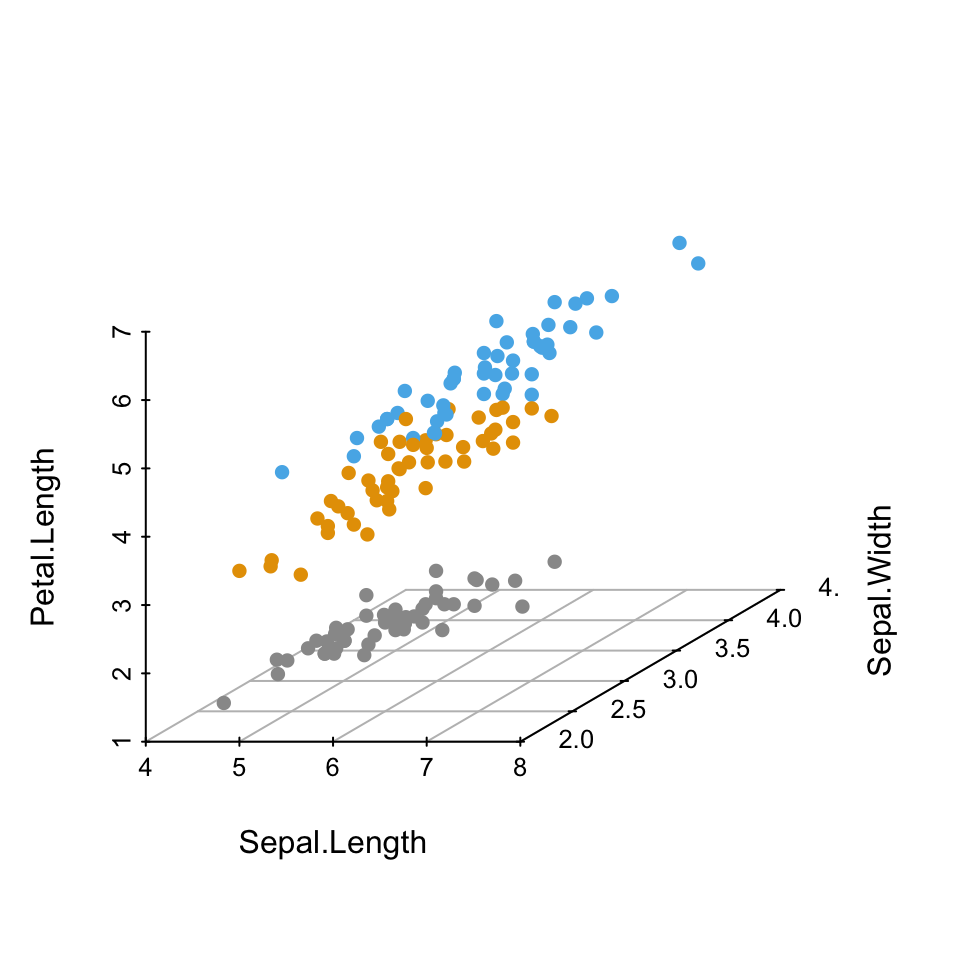# 3D graphics

Previously, we described the essentials of R programming and provided quick start guides for importing data into R as well as visualizing data using R base graphs.

This chapter describes how to create static and interactive three-dimension (3D) graphs. We provide also an R package named graph3d to easily build and customize, step by step, 3D graphs in R software.

# How this chapter is organized?

• Static 3D Scatter Plots
• Interactive 3D Graphs

# Simple 3D Scatter Plots: scatterplot3d Package

• Prepare the data
• The function scatterplot3d()
• Basic 3D scatter plots
• Change the main title and axis labels
• Change the shape and the color of points
• Change point shapes by groups
• Change point colors by groups
• Change the global appearance of the graph
• Remove the box around the plot
• Modification of scatterplot3d output
• Add regression plane and supplementary pointsRead more: —> Simple 3D Scatter Plots: scatterplot3d Package.

# Advanced 3D Graphs: plot3D Package

• Install and load plot3D package
• Prepare the data
• Scatter plots
• Basic scatter plot
• Change the type of the box around the plot
• Change the color by groups
• Change the position of the legend
• 3D viewing direction
• Titles and axis labels
• Tick marks and labels
• Add points and text to an existing plot
• Line plots
• 3D fancy Scatter plot with small dots on basal plane
• Regression plane
• text3D: plot 3-dimensionnal texts
• text3D and scatter3D
• 3D Histogram
• scatter2D: 2D scatter plot
• text2D
• Interactive plot# Interactive 3D Scatter Plots

• Install and load required packages
• Prepare the data
• The function scatter3d
• Basic 3D scatter plots
• Plot the points by groups
• Default plot
• Remove the surfaces
• Change point colors by groups
• Axes
• Change axis labels
• Remove axis scales
• Change axis colors
• Add text labels for the points
• Export images3d scatter plot rgl

Read more: —> Interactive 3D Scatter Plots.

# Guide to RGL 3D Visualization System

• Install the RGL package
• Prepare the data
• Start and close RGL device
• 3D scatter plot
• Basic graph
• Change the background and point colors
• Change the shape of points
• rgl_init(): A custom function to initialize RGL device
• Add a bounding box decoration
• Add axis lines and labels
• Set the aspect ratios of the x, y and z axes
• Change the color of points by groups
• Change the shape of points
• Add an ellipse of concentration
• Regression plane
• Create a movie of RGL scene
• Export images as png or pdf
• Export the plot into an interactive HTML file
• Select a rectangle in an RGL scene
• Identify points in a plot
• R3D Interface
• RGL functions
• Device management
• Shape functions
• Scene management
• Setup the environment
• Appearance setup
• Export screenshot
• Assign focus to an RGL windowRGL movie 3d

Read more —> Guide to RGL 3D Visualization System.

# Infos

This analysis has been performed using R statistical software (ver. 3.2.4).